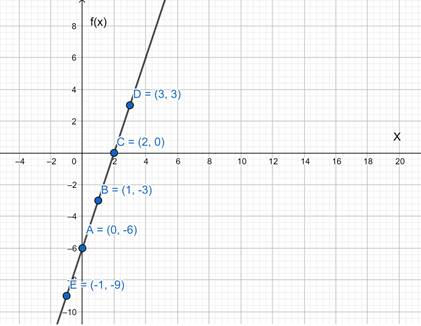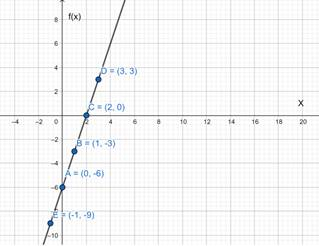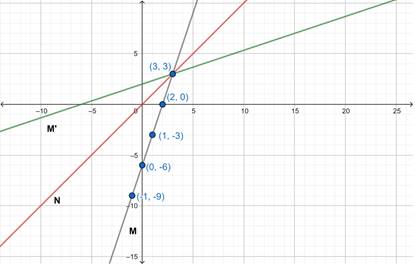# To graph : The function.### Precalculus: Mathematics for Calcu...

6th Edition
Stewart + 5 others
Publisher: Cengage Learning
ISBN: 9780840068071### Precalculus: Mathematics for Calcu...

6th Edition
Stewart + 5 others
Publisher: Cengage Learning
ISBN: 9780840068071

#### Solutions

Chapter 2.7, Problem 61E

a.

To determine

## To graph: The function.

Expert Solution

### Explanation of Solution

Given information:

f(x)=3x6

Graph:

 x 0 1 2 3 −1 f(x) −6 −3 0 3 −9Interpretation: The graph of given function represented a straight line.

b.

To determine

Expert Solution

### Explanation of Solution

Given information:Graph:Interpretation:

Line M is the graph of function f . Line N is the graph of function y=x . After reflecting the line M in line N, we get line M' . Therefore, M' is the graph of function f1 .

c.

To determine

Expert Solution

f1(x)=x+63

### Explanation of Solution

Given information: f(x)=3x6

Concept used: Let, f be a one-to-one function with domain A and range B. Then its inverse function f1 has domain B and range A and is defining by f1(y)=xf(x)=y for any y in B.

Calculation:

First, we replace f(x) with y ,

Then,

f(x)=3x6y=3x6y+6=3xx=y+63f1(y)=y+63f1(x)=x+63

Thus, f1(x)=x+63 .

### Have a homework question?

Subscribe to bartleby learn! Ask subject matter experts 30 homework questions each month. Plus, you’ll have access to millions of step-by-step textbook answers!